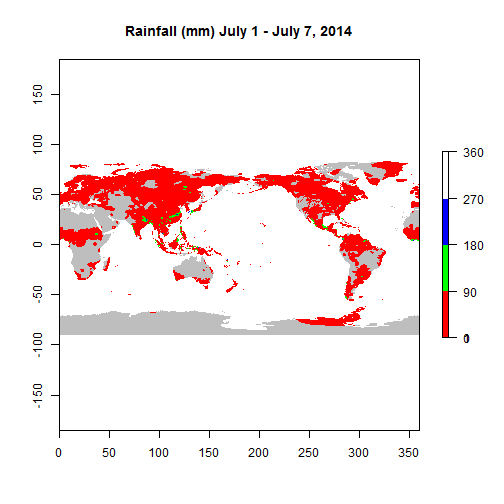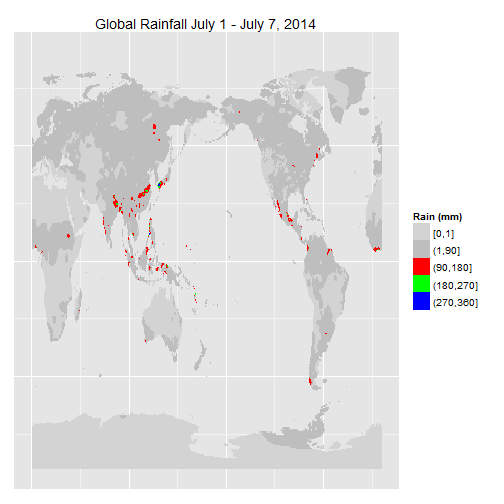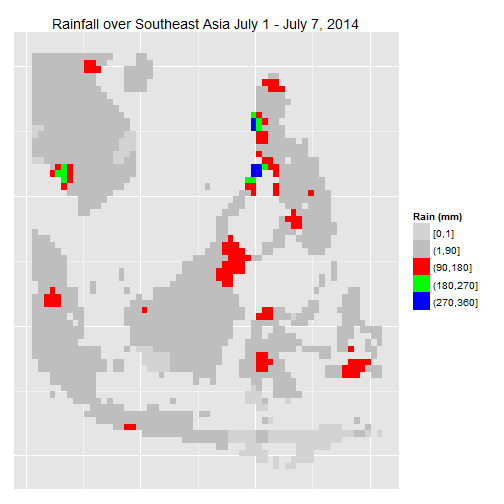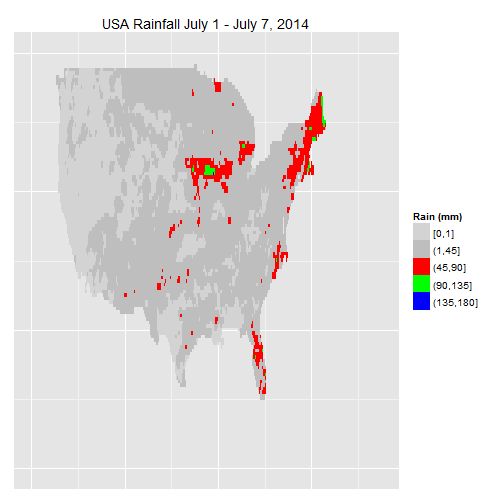Introduction to raincpc

The Climate Prediction Center's (CPC, www.cpc.ncep.noaa.gov) rainfall data for the world (1979 to present, 50 km resolution) and the USA (1948 to present, 25 km resolution), is one of the few high quality, long term, observation based, daily rainfall products available for free. Although raw data is available at CPC's ftp site (ftp.cpc.ncep.noaa.gov/precip/CPC_UNI_PRCP/), obtaining, processing and visualizing the data is not straightforward.

Some issues with the raw CPC data:

• too many files (365/366 files per year, 36 years of global data and 66 years of USA data, separate folder for each year)
• each global file is equivalent to a matrix of 360 rows and 720 columns (for USA specific files, 120 rows and 300 columns)
• file naming conventions have changed over time - one format prior to 2006 and several different formats afterwards
• file formats have changed over time - gzipped files prior to 2008 and plain binary files afterwards
• downloading multiple files simultaneously from the CPC ftp site, for instance using wget, does not seem to work properly
• there is no software readily available to easily process/visualize the data

The package raincpc addresses the above drawbacks by providing functionality to download, process and visualize the raw rainfall data from CPC. Following are some examples.

Using raincpc

After installing the package, load the package along with SDMTools, raster, and ggplot2.

``````library(raincpc)
library(SDMTools)
library(raster)
library(ggplot2)
``````

raincpc comes with the function `cpc_get_rawdata` to download the data from CPC. Following example extracts rainfall data for world for July 1-7 of 2014, during which period the eastern United States was affected by Hurricane Arthur.

``````cpc_get_rawdata(2014, 7, 1, 2014, 7, 7)
``````

Once the data is downloaded, the function `cpc_read_rawdata` can be used to read the binary data and convert it to a raster for analysis and graphics.

``````rain1 <- cpc_read_rawdata(2014, 7, 1)
print(rain1)
``````
``````#> class       : RasterLayer
#> dimensions  : 360, 720, 259200  (nrow, ncol, ncell)
#> resolution  : 0.5, 0.5  (x, y)
#> extent      : 0, 360, -90, 90  (xmin, xmax, ymin, ymax)
#> coord. ref. : NA
#> data source : in memory
#> names       : layer
#> values      : 0, 110.2  (min, max)
``````

Extract the rainfall for all the seven days, and then compute the total rainfall for July 1-7. This rainfall total is used in the following graphics.

``````rain2 <- cpc_read_rawdata(2014, 7, 2)

rain_tot <- rain1 + rain2 + rain3 + rain4 + rain5 + rain6 + rain7
print(rain_tot)
``````
``````#> class       : RasterLayer
#> dimensions  : 360, 720, 259200  (nrow, ncol, ncell)
#> resolution  : 0.5, 0.5  (x, y)
#> extent      : 0, 360, -90, 90  (xmin, xmax, ymin, ymax)
#> coord. ref. : NA
#> data source : in memory
#> names       : layer
#> values      : 0, 336.1  (min, max)
``````

Graphics

Here is the plot of total rainfall for July 1-7 2014.

``````plot(rain_tot,
breaks = c(0, 1, 90, 180, 270, 360),
col = c("grey", "red", "green", "blue"),
main = "Rainfall (mm) July 1 - July 7, 2014")
``````Although it is easy to display the data using the plot method for a RasterLayer, the quality of the graphic is not great. Here is a plot of the same data using ggplot2. First, create a function to convert the data in a ggplot-friendly format.

``````raster_ggplot <- function(rastx) {
require(SDMTools)

stopifnot(class(rastx) == "RasterLayer")

gfx_data <- getXYcoords(rastx)
# lats need to be flipped
gfx_data <- expand.grid(lons = gfx_data\$x, lats = rev(gfx_data\$y),
stringsAsFactors = FALSE, KEEP.OUT.ATTRS = FALSE)
gfx_data\$rain <- rastx@data@values

return (gfx_data)
}
``````

Use the above function to plot the RasterLayer object using ggplot2.

``````rain_gg <- raster_ggplot(rain_tot)

rain_gg\$rain_chunks <- cut(rain_gg\$rain, breaks = c(0, 1, 90, 180, 270, 360),
include.lowest = TRUE)

gfx_gg <- ggplot(data = rain_gg)
gfx_gg <- gfx_gg + geom_raster(aes(lons, lats, fill = rain_chunks))
gfx_gg <- gfx_gg + scale_fill_manual(values = c("lightgrey", "grey", "red", "green", "blue"))
gfx_gg <- gfx_gg + theme(axis.text = element_blank(), axis.ticks = element_blank())
gfx_gg <- gfx_gg + labs(x = NULL, y = NULL, fill = "Rain (mm)")
gfx_gg <- gfx_gg + ggtitle("Global Rainfall July 1 - July 7, 2014")

plot(gfx_gg)
``````Extracting Regional Rainfall

When rainfall over other regions of the world is desired, SDMTools could be used. SDMTools has functionality to extract regional data from the RasterLayer object. In order to extract the data, say over Southeast Asia, specify a lat-lon bounding box as a data frame and extract the data for this box.

``````lon_vals <- seq(100.75, 130.75, 0.5)
lat_vals <- seq(-10.75, 20.75, 0.5)
reg_box <- expand.grid(lons = lon_vals, lats = lat_vals,
stringsAsFactors = FALSE, KEEP.OUT.ATTRS = FALSE)
reg_box\$rain <- extract.data(reg_box, rain_tot)
reg_box\$rain_chunks <- cut(reg_box\$rain, breaks = c(0, 1, 90, 180, 270, 360),
include.lowest = TRUE)
``````

Use ggplot to plot the regional rainfall over Southeast Asia.

``````gfx_gg <- ggplot(data = reg_box)
gfx_gg <- gfx_gg + geom_raster(aes(lons, lats, fill = rain_chunks))
gfx_gg <- gfx_gg + scale_fill_manual(values = c("lightgrey", "grey", "red", "green", "blue"))
gfx_gg <- gfx_gg + theme(axis.text = element_blank(), axis.ticks = element_blank())
gfx_gg <- gfx_gg + labs(x = NULL, y = NULL, fill = "Rain (mm)")
gfx_gg <- gfx_gg + ggtitle("Rainfall over Southeast Asia July 1 - July 7, 2014")

plot(gfx_gg)
``````Extracting Rainfall only for the USA

The default options in raincpc were set to extract and analyze data for the whole world. However, CPC also provides higher resolution (25 km instead of 50 km for the world) rainfall data for the USA. The raincpc package could be used with the flag `usa = TRUE` for USA-specific data.

Similar to the above example, rainfall data for the USA for July 1-7 of 2014 is extracted below.

``````cpc_get_rawdata(2014, 7, 1, 2014, 7, 7, usa = TRUE)
rain1 <- cpc_read_rawdata(2014, 7, 1, usa = TRUE)
print(rain1)
``````
``````#> class       : RasterLayer
#> dimensions  : 120, 300, 36000  (nrow, ncol, ncell)
#> resolution  : 0.25, 0.25  (x, y)
#> extent      : 230, 305, 20, 50  (xmin, xmax, ymin, ymax)
#> coord. ref. : NA
#> data source : in memory
#> names       : layer
#> values      : 0, 120.1  (min, max)
``````
``````rain2 <- cpc_read_rawdata(2014, 7, 2, usa = TRUE)
rain3 <- cpc_read_rawdata(2014, 7, 3, usa = TRUE)
rain4 <- cpc_read_rawdata(2014, 7, 4, usa = TRUE)
rain5 <- cpc_read_rawdata(2014, 7, 5, usa = TRUE)
rain6 <- cpc_read_rawdata(2014, 7, 6, usa = TRUE)
rain7 <- cpc_read_rawdata(2014, 7, 7, usa = TRUE)

rain_tot <- rain1 + rain2 + rain3 + rain4 + rain5 + rain6 + rain7
print(rain_tot)
``````
``````#> class       : RasterLayer
#> dimensions  : 120, 300, 36000  (nrow, ncol, ncell)
#> resolution  : 0.25, 0.25  (x, y)
#> extent      : 230, 305, 20, 50  (xmin, xmax, ymin, ymax)
#> coord. ref. : NA
#> data source : in memory
#> names       : layer
#> values      : 0, 137.7  (min, max)
``````
``````rain_gg <- raster_ggplot(rain_tot)

rain_gg\$rain_chunks <- cut(rain_gg\$rain, breaks = c(0, 1, 45, 90, 135, 180),
include.lowest = TRUE)

gfx_gg <- ggplot(data = rain_gg)
gfx_gg <- gfx_gg + geom_raster(aes(lons, lats, fill = rain_chunks))
gfx_gg <- gfx_gg + scale_fill_manual(values = c("lightgrey", "grey", "red", "green", "blue"))
gfx_gg <- gfx_gg + theme(axis.text = element_blank(), axis.ticks = element_blank())
gfx_gg <- gfx_gg + labs(x = NULL, y = NULL, fill = "Rain (mm)")
gfx_gg <- gfx_gg + ggtitle("USA Rainfall July 1 - July 7, 2014")

plot(gfx_gg)
``````Writing Output

The extracted rainfall data could be saved by setting the `write_output` flag to TRUE. The `write.asc` and related functions from SDMTools package could also be used to write the RasterLayer to output.

``````rain1 <- cpc_read_rawdata(2014, 7, 1, usa = TRUE, write_output = TRUE)
``````